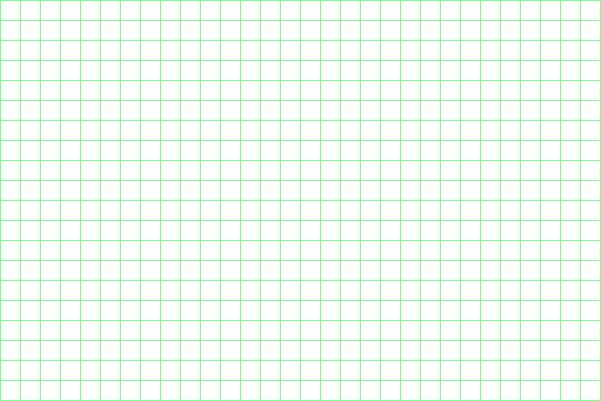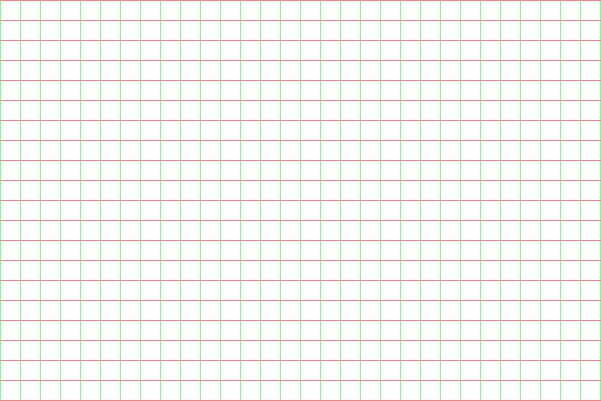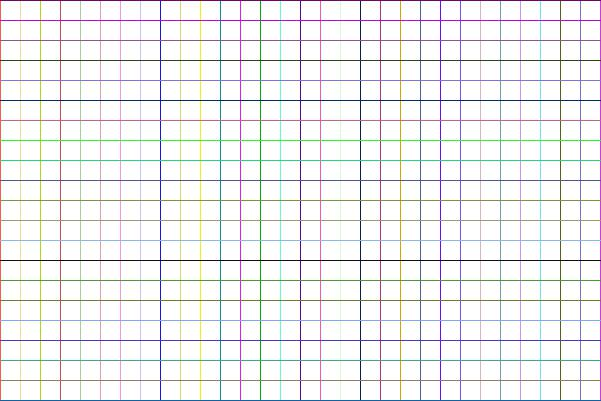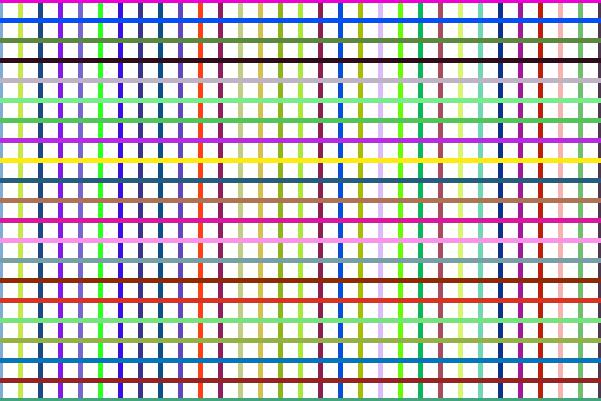# Drawing graphic lines using PHP GD Support

``imageline(\$image,\$x1,\$y1,\$x2,\$y2,\$color)``
 `\$image` Image created using ImageCreate() or imagecreatetruecolor() `\$x1` X coordinate of start point ( X : Horizontal ) `\$y1` Y coordinate of start point ( Y : Vertical) `\$x2` X coordinate of end point ( X : Horizontal ) `\$y2` Y coordinate of end point ( Y : Vertical ) `\$color` Color of the line by using imagecolorallocate().
If you have installed GD library then let us try to draw some lines in an image using gd support. We will open our file in a new window as we have to tell browser ( through header ) that we are sending image.

Using gd we will first learn how to draw line. We will learn some basic functions used in creating and managing images.
``\$im = @ImageCreate (100, 200)``
The above line creates and image identifier of width 100 and height 200. The top left coordinate is set as 0, 0

Drawing lines and patterns using different thickness and colours using imageline() in PHP GD
``\$background_color = ImageColorAllocate (\$im, 234, 234, 234);``
Background color is set here for the image with the identifier \$im. Here we have set it to a light gray background. The color of the background can be changed by changing the values of red, green, blue ( now all are set to 234 to create light gray background)

Same way let us set a text color for the image
``````\$text_color = ImageColorAllocate (\$im, 233, 14, 91);
imageline (\$im,\$x1,\$y1,\$x2,\$y2,\$text_color);``````
The above line will show the line from where to where it is to be drawn. Staring from \$x1,\$x2 to \$x2, \$y2. Note that top left is at the coordinate 0,0. The lines below will give how the line will appear with different values of the coordinates used in imageline function.

Now with this image, let us draw
``ImagePng (\$im);``
With the above knowledge, you can see that the command imageline (\$im,\$x1,\$y1,\$x2,\$y2,\$text_color) draws lines between two points defined with two coordinates. To learn the different images we get ( or different lines ) with the change in value of coordinates ( x and y ) we will list a set of values and corresponding image link. Click the link right of the coordinates to check what image will be drawn with the value given at left. All images will be opened in a new window.

 imageline (\$im,0,0,50,100,\$text_color) Display imageline (\$im,0,0,100,100,\$text_color) Display imageline (\$im,100,0,0,200,\$text_color) Display imageline (\$im,0,0,100,200,\$text_color) Display

With above example you can see how the coordinates affect the line inside the image. Now let us dray two lines in the same image one after the other.

 imageline (\$im,0,0,100,200,\$text_color); imageline (\$im,100,0,0,200,\$text_color); Display

Above examples must have given you some idea on how to draw lines using gd support in PHP. You can use the forum if you have any doubt. Now here you can see the code we used to draw the lines in small window. Note that this code has two imageline functions to draw two lines. Change the data and see how the line changes.

``````<?php
\$x1=\$_GET['x1'];
\$x2=\$_GET['x2'];
\$y1=\$_GET['y1'];
\$y2=\$_GET['y2'];

\$im = @ImageCreate (100, 200)
or die ("Cannot Initialize new GD image stream");
\$background_color = ImageColorAllocate (\$im, 224, 234, 234);
\$text_color = ImageColorAllocate (\$im, 233, 14, 91);
// imageline (\$im,\$x1,\$y1,\$x2,\$y2,\$text_color);
imageline (\$im,0,0,100,200,\$text_color);
imageline (\$im,100,0,0,200,\$text_color);
ImagePng (\$im);
?>``````
Save the above code in a file and open it without adding any html code or data to it. With this learning on how to draw lines we will move to our next learning.

## Example

Note that PHP counts from 0, so if we take width as 600 then for the last line when `\$i=600` can't be drawn at the edge of the image so we kept width and height as 601 and 401. You can experiment this by changing width height to 600 and 400.``````<?Php
\$im = @ImageCreate (601, 401) // added one pixel for border
or die ("Cannot Initialize new GD image stream");
\$gap=20; // change this value to increase the lines
\$background_color = ImageColorAllocate (\$im, 255, 255, 255);
\$text_color = ImageColorAllocate (\$im, 128, 255, 136);
//imagesetthickness(\$im, 5);
for(\$i=0;\$i<=600;\$i +=\$gap){
imageline (\$im,\$i,0,\$i,400,\$text_color);
}
for(\$i=0;\$i<=400;\$i +=\$gap){
imageline (\$im,0,\$i,600,\$i,\$text_color);
}
Imagejpeg (\$im);
imagedestroy(\$im); //memory is removed.
?>``````
We can use different color for horizontal lines``````header ("Content-type: image/jpeg");
\$im = @ImageCreate (601, 401) // added one pixel for border
or die ("Cannot Initialize new GD image stream");
\$size=20; // change this value to increase the lines
\$background_color = ImageColorAllocate (\$im, 255, 255, 255);
\$text_color = ImageColorAllocate (\$im, 128, 255, 136);
//imagesetthickness(\$im, 5);
// vertical lines
for(\$i=0;\$i<=600;\$i +=\$size){
imageline (\$im,\$i,0,\$i,400,\$text_color);
}
// Horizontal lines
\$text_color = ImageColorAllocate (\$im, 255, 128, 128);
for(\$i=0;\$i<=400;\$i +=\$size){
imageline (\$im,0,\$i,600,\$i,\$text_color);
}
Imagejpeg (\$im);
imagedestroy(\$im); //memory is removed. ``````
By using random number generator rand(min,max) we can create all lines in different colours.``````header ("Content-type: image/jpeg");
\$im = @ImageCreate (601, 401) // added one pixel for border
or die ("Cannot Initialize new GD image stream");
\$size=20; // change this value to increase the lines
\$background_color = ImageColorAllocate (\$im, 255, 255, 255);

for(\$i=0;\$i<=600;\$i +=\$size){
\$r=rand(1,255);
\$g=rand(1,255);
\$b=rand(1,255);
\$text_color = ImageColorAllocate (\$im, \$r, \$g,\$b);
imageline (\$im,\$i,0,\$i,400,\$text_color);
}
// Horizontal lines

for(\$i=0;\$i<=400;\$i +=\$size){
\$r=rand(1,255);
\$g=rand(1,255);
\$b=rand(1,255);
\$text_color = ImageColorAllocate (\$im, \$r, \$g,\$b);
imageline (\$im,0,\$i,600,\$i,\$text_color);
}
Imagejpeg (\$im);
imagedestroy(\$im); //memory is removed. ``````

## Managing thickness of linesAdd this line before the for loop in above code.
``imagesetthickness(\$im, 5);``
.Subscribe to our YouTube Channel here

## Subscribe

* indicates required
Subscribe to plus2netplus2net.com

GD functions in PHP for handling images & graphics

How to check GD support in PHP
Header for an image in browser
Drawing of graphic lines using GD
Plotting line graph by drawing data from table
Adding vertical grid to line graph
Imagearc drawing arc and circle
Imagefilledarc drawing filled arc and circle
Resizing and adding border to Images
Script for Watermarking image on the fly using PHP GD
Generate image by using user input form data